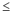# Specify how to estimate the parameters for I-MR Chart

Stat > Control Charts > Variables Charts for Individuals > I-MR > I-MR Options > Estimate

## Specify observations to estimate parameters

If some observations have erratic data because of special causes that you have corrected, you can omit these observations from the calculations to avoid incorrect parameter estimates.

1. From the drop-down list, specify whether you want to list the observations to omit, or the observations to include.
• To list the observations to omit, select Omit the following subgroups when estimating parameters (eg, 3 12:15).
• To list the observations to include, select Use the following subgroups when estimating parameters (eg, 3 12:15).
2. List the observations. Use a colon to indicate a range of observations. Leave a space between each observation or range of observations.
For example, to specify observations 2, 9, 44, 45, 46, and 47, enter 2 9 44:47.

## Method for estimating standard deviation

Choose the method to estimate the standard deviation that Minitab uses to calculate the control limits.

Subgroup size = 1
• Average moving range: The average moving range is the average value of the moving range of two or more consecutive points. Use this method when the subgroup size is 1.
• Median moving range: The median moving range is the median value of the moving range of two or more consecutive points. Use this method when the data have extreme ranges that influence average of the moving ranges.

## Specify length of moving range

In Length of moving range, enter the number of observations that are used to calculate the moving range. By default, a length of 2 is used because consecutive values have the greatest chance of being alike. The length must be100.

If the data are cyclical, consider using a moving range of a different length. For example, if you collect quarterly data, consider using a moving range of length 4 to ensure that one observation from each season is included in the calculation.

## Use Nelson estimate

Select Use Nelson estimate to correct for unusually large moving range values in the calculation of the control limits. The procedure is similar to the procedure proposed by Nelson1. Minitab eliminates any moving range values that are more than 3 standard deviations larger than the average moving range, then recalculates the average moving range and the control limits.
1 L. S. Nelson (1982). "Control Charts for Individual Measurements," Journal of Quality Technology, 14, 172−173.